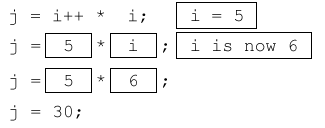# Post Increment Operator in a Java Expression

In this article we’ll see how the post increment operator works and how it behaves when we use it in a complex expression. First lets see a very simple case where we use post increment operator with assignment,

int i = 5;
int j = i++;
System.out.print("i="+i);
System.out.print(", j="+j);

The output of the above code will be “i=6, j=5”. The reason for this output is that first `i` was used in the expression and then its value was incremented.

An expression is is any calculation or method call or both which returns a value assignable to a variable.

In our code, `i++` was an expression. An expression can be more complicated or simpler than that. Like `i + j - 2 / z` is also an expression and just plain `i` is also an expression (because you can assign the value of `i` to another variable like `int j = i;`).

In our code example above, first `i`‘s value was used in the expression, and then it was incremented. And at the end the result of the expression was assigned to `j`. So `int j=i++` first became `int j = 5` (the assignment hasn’t happened yet), then the value of `i` was incremented and finally 5 was assigned to `j`. Thus, when we use the post increment operator in an expression, first the old value of the variable is used in the expression and then the variable’s value is incremented. Remember that the assignment happens after the value of `i` is incremented.

When the JVM executes an expression, it kind of sees each variable in the expression as a fill in the blank. The value of the variable is put in the blank to complete the expression. So if we have a code like this

int i = 5, j = 6, k = 7;
int a = i + j + k;

To solve the expression, the compiler will replace `i`, `j` and `k` with their actual values. It goes something like this,When we used the post increment operator in the expression, the fill in the blank got filled before `i` was incremented.

Lets take a look at another example of assignment with prefix increment operator

int i = 5;
int j = i++ * 10;
System.out.print("i="+i);
System.out.print(", j="+j);

Here the expression is little more complicated. We’ve added a multiplication to the scenario. The result of this code won’t be very surprising, it will be “i=6, j=50”. This shouldn’t be hard to understand. Here again, the value of `i` in the expression is 5 and it is incremented after that. 5 is multiplied by 10 and then assigned to `j`. Actually we introduced this code only as an intermediate step to our next code. In our next code, we’ll see what happens if we use `i` twice in an expression. As we know our expression is evaluated from left to right. So what happens if we increment `i` and then again use it in the right part of the expression. Lets see this in code,

int i = 5;
int j = i++ * i;
System.out.print("i="+i);
System.out.print(", j="+j);

This is where it gets a little tricky. The output of this code will be “i=6, j=30”. This means that the expression resolved to `j = 5 * 6`. How did this happen? Well first the left side of the multiplication is solved i.e. `i++` is calculated, the old value of `i` i.e. 5 is used in the expression and then `i` is incremented. After that the right side of the multiplication is solved, so the new value of `i` i.e. 6 is used. After that 5 and 6 are multiplied and the result assigned to `j`.As you can see in the diagram above, the first box is filled with the old value of `i` and after that `i` is incremented. Then the new value of `i` is used in the second box in the expression.

If you are wondering why is ++ evaluated before * and the assignment happens in the end, this is due to operator precedence. Increment operators have a higher precedence then multiplication, division, addition etc. Assignment i.e. = operator has very low precedence, so the assignment happens in the end.

There is another case of using assignment with post increment operator which might look strange at first. Lets have a look,

int i = 0;
i = i++;
System.out.print("i="+i);

Now the output of this code will be “i=0”. You might wonder why didn’t the value of `i` got incremented. Actually it did got incremented. What happened here was when `i++` was solved, the old value of `i` i.e. 0 was used in the expression. Then the value of `i` was incremeted to 1. But at the end, the assignment happened so 0 was assigned to `i`. Because of this it seems as if the value of `i` was never incremented.

That’s it for this tutorial, we hope you found it helpful.

This is an oversimplified definition of an expression. Remember that an expression doesn’t necessarily have to return a value, it can be void too.